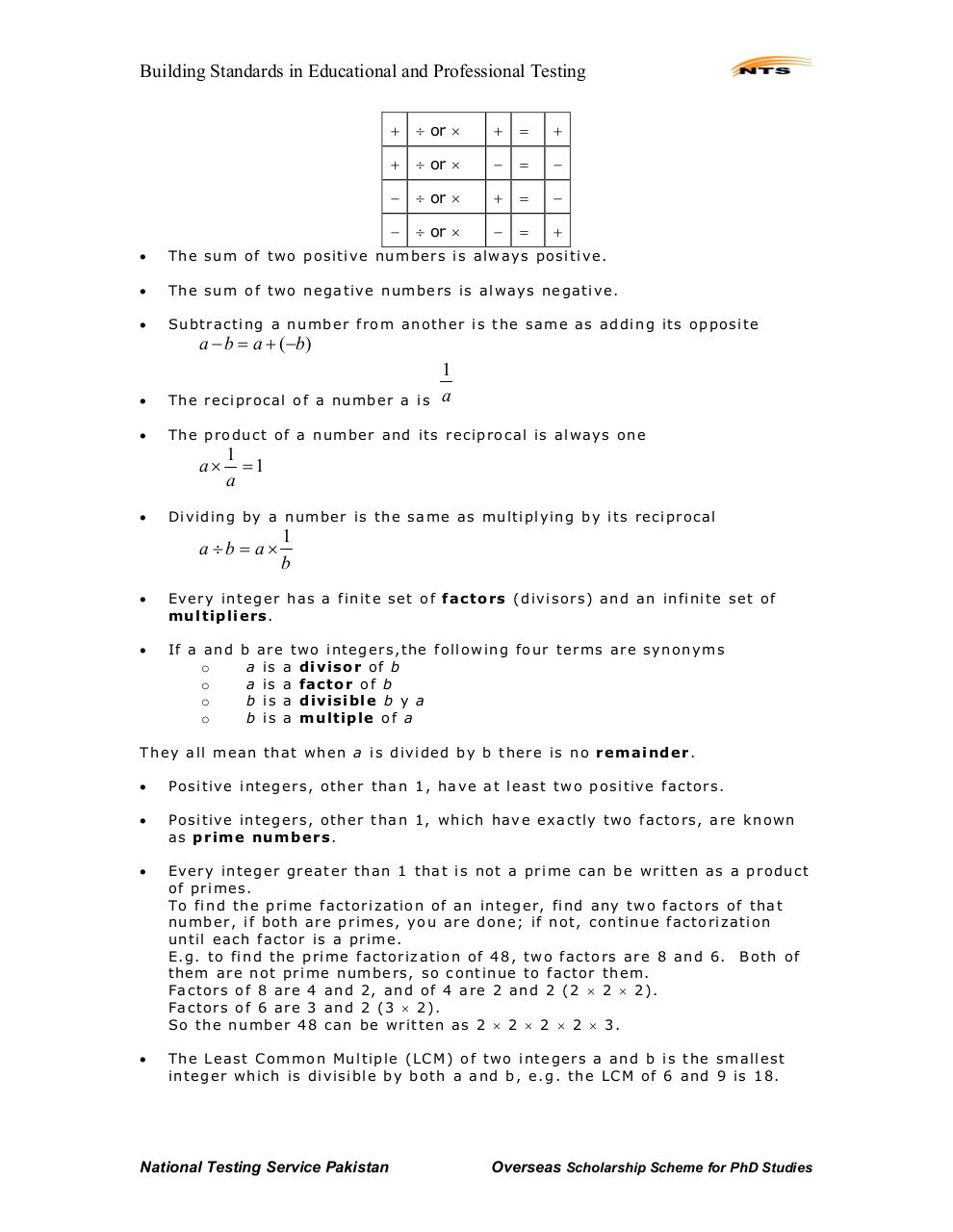# NTS Book for GAT General.pdfPage 1 2 3 456142

#### Text preview

Building Standards in Educational and Professional Testing
+

÷ or ×

+

=

+

+

÷ or ×

=

÷ or ×

+

=

÷ or ×

=

+

The sum of two posi tive numbers i s al ways posi ti ve.

The sum of two negative numbers i s al ways negati ve.

S u btracti ng a number from another i s the same as ad di ng i ts opposi te

a − b = a + (−b)

1
a

The reci pro cal of a number a i s

The produc t of a number and i ts reci procal i s al ways one

1
=1
a

D iv i di n g by a n u m ber i s th e same as m u l ti pl yi n g by i ts reci procal

a ÷b = a×

1
b

Every i n teger has a fini te set of factor s (di v i sors) an d an i nfi ni te set of
m u l t i p l i e r s.

If a and
o
o
o
o

b are two i n tegers, the f oll owi n g four terms are synonyms
a i s a divisor o f b
a i s a factor o f b
b i s a divisible b y a
b i s a multiple o f a

They al l mean that when a i s divi ded by b there i s no r e m a i n der .

Posi ti ve i n tegers, other than 1, have at l east two posi tive factors.

Posi ti ve i n tegers, other than 1, whi ch have exactl y two factors, are known
as p r i m e nu m b e r s.

Every i n teger greater than 1 that i s not a pri m e can be wri tten as a product
of pri m es.
T o f i n d t h e p r i m e f a c to r i z a ti o n of a n i n t e g e r , fi n d a n y t w o f a c t o r s o f t h a t
number, i f both are pr i m es, you are done; i f not, continue factorizati on
until each factor i s a pri m e.
E. g. to fi nd the pri m e factori z ati on of 48, two factors are 8 and 6. Both of
them are not pri m e numbers, so conti nue to factor them.
Factors of 8 are 4 and 2, and of 4 are 2 and 2 (2 × 2 × 2).
Factors of 6 are 3 and 2 (3 × 2).
S o the number 48 ca n be wri tten as 2 × 2 × 2 × 2 × 3.

Th e Leas t C om m on Mu l ti pl e (LCM) of tw o in tegers a a n d b i s th e sm all est
i n teger which i s di vi sibl e by both a and b, e. g. the LCM of 6 and 9 i s 18.

National Testing Service Pakistan

Overseas Scholarship Scheme for PhD Studies